﻿ 永磁同步电机的转子初始位置检测

# 永磁同步电机的转子初始位置检测Rotor Initial Position Detection of Permanent Magnet Synchronous Motor

Abstract: Aiming at poor reliability and small applicable range of the traditional rotor initial position detection method for permanent magnet synchronous motor, a rotor position detection method using high frequency signal injection is improved. The high frequency signal is injected in the three-phase permanent magnet synchronous motor. The salient effect based on the current con-taining rotor position information is obtained and filtered, where a band-pass filter based on proportional resonance controller is designed, which simplifies the structure of the filter and is easy to realize digitally. Then, the position information is extracted by a heterodyne method and the position is accurately estimated by the rotor position observer. The simulation results show that the presented method can accurately estimate the rotor position and the average error of position detection is within 0.1˚.

1. 引言

2. 高频激励下三相永磁同步电机的数学模型

$\begin{array}{c}{u}_{d}=R{i}_{d}+\frac{\text{d}}{\text{d}t}{\psi }_{d}-{\omega }_{e}{\psi }_{q}\\ {u}_{q}=R{i}_{q}+\frac{\text{d}}{\text{d}t}{\psi }_{q}+{\omega }_{e}{\psi }_{d}\end{array}$ (1)

$\left\{\begin{array}{c}{\psi }_{d}={L}_{d}{i}_{d}+{\psi }_{f}\\ {\psi }_{q}={L}_{q}{i}_{q\begin{array}{c}\end{array}}\begin{array}{cc}& \end{array}\end{array}$ (2)

$\left\{\begin{array}{c}{u}_{d}=R{i}_{d}+{L}_{d}\frac{\text{d}}{\text{d}t}{i}_{d}-{\omega }_{e}{L}_{q}{i}_{q}\begin{array}{cc}\begin{array}{cc}& \end{array}& \end{array}\\ {u}_{q}=R{i}_{q}+{L}_{q}\frac{\text{d}}{\text{d}t}{i}_{q}+{\omega }_{e}\left({L}_{d}{i}_{d}+{\psi }_{f}\right)\end{array}$ (3)

$\left[\begin{array}{c}{u}_{ds}\\ {u}_{qs}\end{array}\right]=R\left[\begin{array}{c}{i}_{ds}\\ {i}_{qs}\end{array}\right]+\frac{\text{d}}{\text{d}t}\left[\begin{array}{c}{\psi }_{ds}\\ {\psi }_{qs}\end{array}\right]$ (4)

$\left[\begin{array}{c}{\psi }_{ds}\\ {\psi }_{qs}\end{array}\right]=\left[\begin{array}{cc}L+\Delta L\mathrm{cos}2{\theta }_{r}& \Delta L\mathrm{sin}2{\theta }_{r}\\ \Delta L\mathrm{sin}2{\theta }_{r}& L-\Delta L\mathrm{cos}2{\theta }_{r}\end{array}\right]\left[\begin{array}{c}{i}_{ds}\\ {i}_{qs}\end{array}\right]+{\psi }_{f}\left[\begin{array}{c}\mathrm{cos}{\theta }_{r}\\ \mathrm{sin}{\theta }_{r}\end{array}\right]$ (5)

$\Delta L=\left({L}_{d}-{L}_{q}\right)/2$ —半差电感。

${L}_{dqs}=\left[\begin{array}{cc}L+\Delta L\mathrm{cos}2{\theta }_{r}& \Delta L\mathrm{sin}2{\theta }_{r}\\ \Delta L\mathrm{sin}2{\theta }_{r}& L-\Delta L\mathrm{cos}2{\theta }_{r}\end{array}\right]$ (6)

$\left\{\begin{array}{c}{u}_{dhs}\approx {L}_{d}\frac{\text{d}{i}_{dhs}}{\text{d}t}\\ {u}_{qhs}\approx {L}_{q}\frac{\text{d}{i}_{qhs}}{\text{d}t}\end{array}$ (7)

$\left\{\begin{array}{c}{T}_{e}=\frac{2}{3}{n}_{p}\left({\psi }_{d}{i}_{d}-{\psi }_{q}{i}_{q}\right)\\ \frac{Jp{\omega }_{e}}{{n}_{p}}={T}_{e}-{T}_{L}-{R}_{\Omega }\frac{{\omega }_{e}}{{n}_{p}}\end{array}$ (8)

3. 基于高频信号注入法的转子位置估计

3.1. 高频电压信号的选择

3.2. 基于比例谐振控制器的带通滤波器设计

3.2.1. 比例谐振控制原理

${G}_{PR}{\left(s\right)}_{1}=\frac{1}{2}\left[{G}_{PI}\left(s+j{\omega }_{0}\right)+{G}_{PI}\left(s-j{\omega }_{0}\right)\right]={K}_{p}+\frac{2{K}_{i}s}{s+{\omega }_{0}^{2}}$ (9)Figure 1. Quasi-PR controller block diagram

${G}_{PR}\left(s\right)={K}_{p}+\frac{2{K}_{i}{\omega }_{c}s}{{s}^{2}+2{\omega }_{c}s+{\omega }_{0}^{2}}$ (10)

3.2.2. 比例谐振带通滤波器设计方法

$s=\frac{{\omega }_{0}\left(z-1\right)}{\mathrm{tan}\left({\omega }_{0}{T}_{s}/2\right)\left(z+1\right)}$ (11)

${G}_{PR}\left(z\right)=\frac{{b}_{0}+{b}_{1}z+{b}_{2}{z}^{2}}{{a}_{0}+{a}_{1}z+{a}_{2}{z}^{2}}$ (12)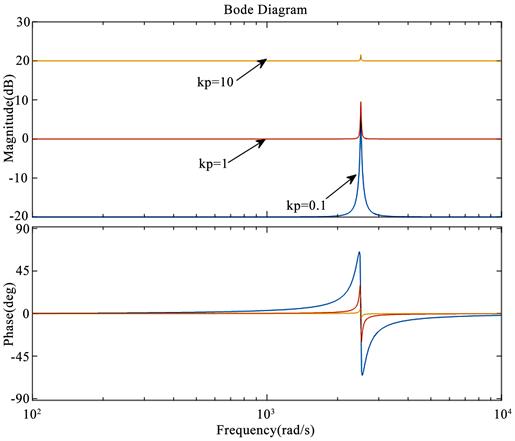Figure 2. The result of changing ${K}_{p}$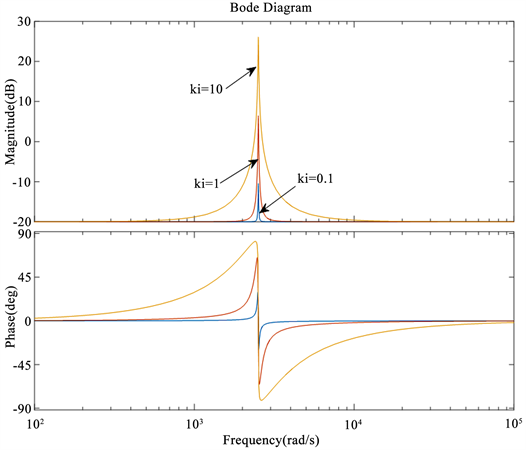Figure 3. The result of changing ${K}_{i}$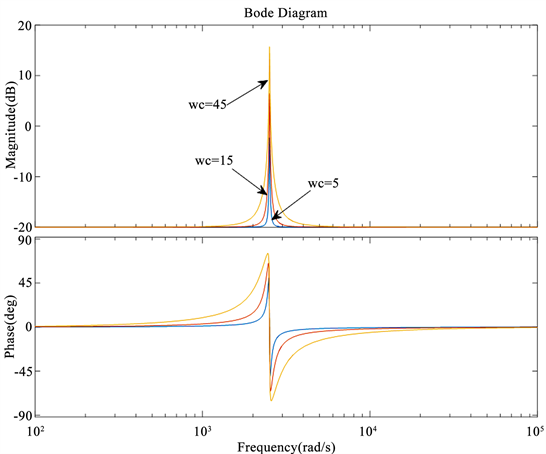Figure 4. The result of changing ${\omega }_{c}$

$\begin{array}{l}{b}_{0}=\frac{-d{k}_{i}{\omega }_{c}}{{d}^{2}+d{\omega }_{c}+{\omega }_{0}^{2}},\text{\hspace{0.17em}}\text{\hspace{0.17em}}\text{\hspace{0.17em}}{b}_{2}=\frac{d{k}_{i}{\omega }_{c}}{{d}^{2}+d{\omega }_{c}+{\omega }_{0}^{2}},\text{\hspace{0.17em}}\text{\hspace{0.17em}}\text{\hspace{0.17em}}{b}_{1}=0\\ {a}_{0}=\frac{{d}^{2}-d{\omega }_{c}+{\omega }_{0}^{2}}{{d}^{2}+d{\omega }_{c}+{\omega }_{0}^{2}},\text{\hspace{0.17em}}\text{\hspace{0.17em}}\text{\hspace{0.17em}}{a}_{1}=\frac{2\left({\omega }_{c}^{2}-{d}^{2}\right)}{{d}^{2}+d{\omega }_{c}+{\omega }_{0}^{2}},\text{\hspace{0.17em}}\text{\hspace{0.17em}}\text{\hspace{0.17em}}{a}_{2}=1\end{array}$

$y\left(k\right)={b}_{0}x\left(k-2\right)+{b}_{2}x\left(k\right)-{a}_{1}y\left(k-1\right)-{a}_{0}y\left(k-2\right)$ (13)

3.3. 旋转高频电压激励下永磁同步电机的电流响应

${u}_{\alpha \beta hs}=\left[\begin{array}{c}{u}_{\alpha hs}\\ {u}_{\beta hs}\end{array}\right]=\left[\begin{array}{c}{V}_{in}\mathrm{cos}{\omega }_{in}t\\ {V}_{in}\mathrm{sin}{\omega }_{in}t\end{array}\right]={V}_{in}{\text{e}}^{j{\omega }_{in}t}$ (14)

${u}_{dqhs}={u}_{\alpha \beta hs}{e}^{-j{\theta }_{r}}={V}_{in}{\text{e}}^{j{\omega }_{in}t}$ (15)

$\begin{array}{l}{i}_{dqhs}=\frac{{V}_{in}}{{L}_{d}}\int \mathrm{cos}\left({\omega }_{in}t-{\theta }_{r}\right)\text{d}t+j\frac{{V}_{in}}{{L}_{q}}\\ \begin{array}{ccc}& =& \frac{{V}_{in}}{{\omega }_{in}{L}_{d}{L}_{q}}\end{array}\left[\frac{{L}_{d}+{L}_{q}}{2}{\text{e}}^{j\left({\omega }_{in}t-{\theta }_{r}-\frac{\text{π}}{\text{2}}\right)}+\frac{{L}_{d}-{L}_{q}}{2}{\text{e}}^{j\left(-{\omega }_{in}t+{\theta }_{r}+\frac{\text{π}}{\text{2}}\right)}\right]\end{array}$ (16)

${i}_{\alpha \beta hs}={i}_{dq}{e}^{j{\theta }_{r}}={I}_{cp}{\text{e}}^{j\left({\omega }_{in}t-\frac{\text{π}}{2}\right)}+{I}_{cn}{\text{e}}^{j\left(-{\omega }_{in}t+2{\theta }_{r}+\frac{\text{π}}{2}\right)}$ (17)

${I}_{cn}$ —负相序高频电流分量的幅值， ${I}_{cn}=\frac{{V}_{in}}{{\omega }_{in}{L}_{d}{L}_{q}}\frac{{L}_{d}-{L}_{q}}{2}$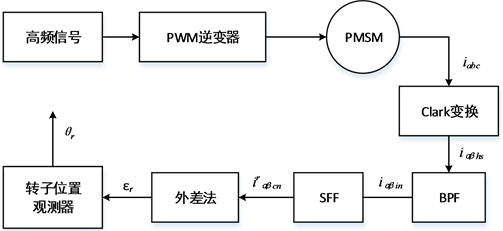Figure 5. Corresponding current response system diagram in the motor

3.4. 转子位置估计的实现方法

${i}_{dqhs_n}={I}_{cn}{\text{e}}^{j\left(-{\omega }_{in}t+2{\theta }_{r}+\frac{\text{π}}{2}\right)}$ (18)

$\begin{array}{l}\epsilon ={i}_{qhs_n}\mathrm{cos}\left(2{\stackrel{^}{\theta }}_{r}-{\omega }_{in}t+\frac{\text{π}}{\text{2}}\right)-{i}_{dhs_n}\mathrm{sin}\left(2{\stackrel{^}{\theta }}_{r}-{\omega }_{in}t+\frac{\text{π}}{\text{2}}\right)\\ \begin{array}{c}\end{array}={I}_{cn}\mathrm{sin}\left(2{\theta }_{r}-2{\stackrel{^}{\theta }}_{r}\right)\end{array}$ (19)

4. 仿真建模与结果分析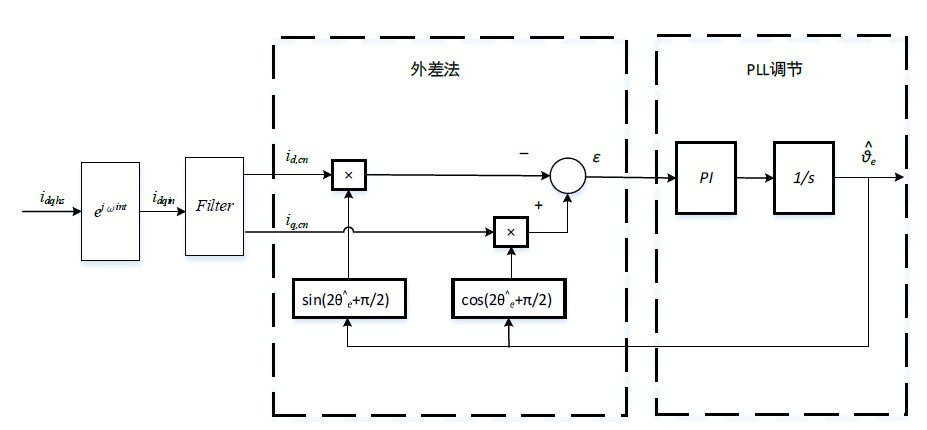Figure 6. Rotor position detection schematic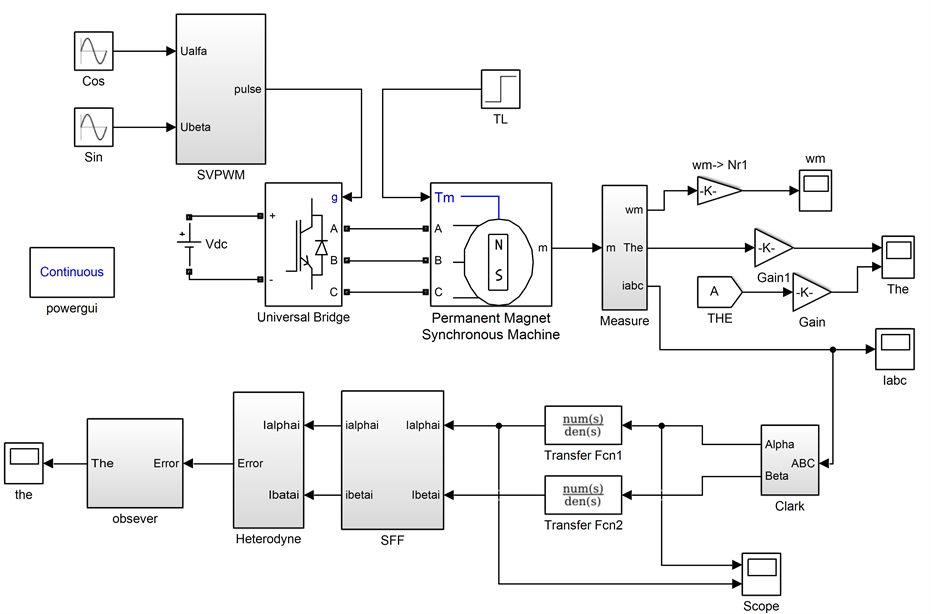Figure 7. Three-phase PMSM initial position detection simulation of rotating high-frequency voltage signal injectionTable 1. Simulation PMSM parameter table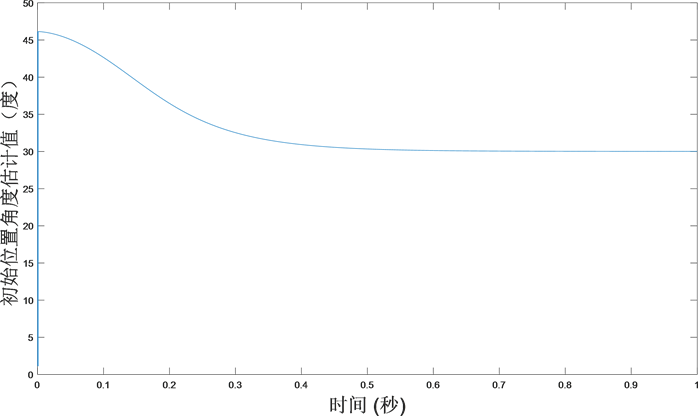Figure 8. Simulation result of PMSM at 30˚ initial position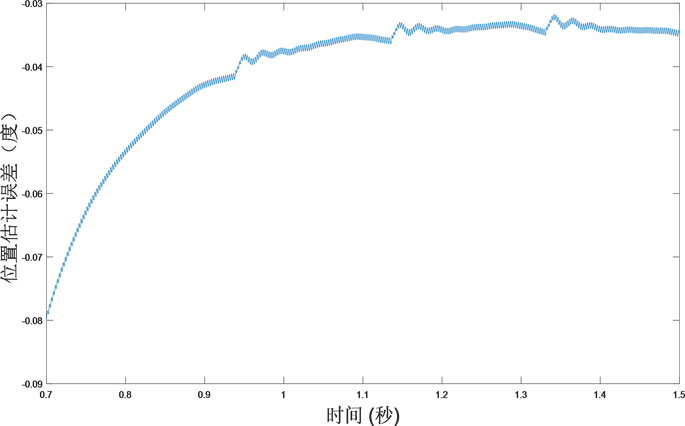Figure 9. Position error at a PMSM initial angle of 30˚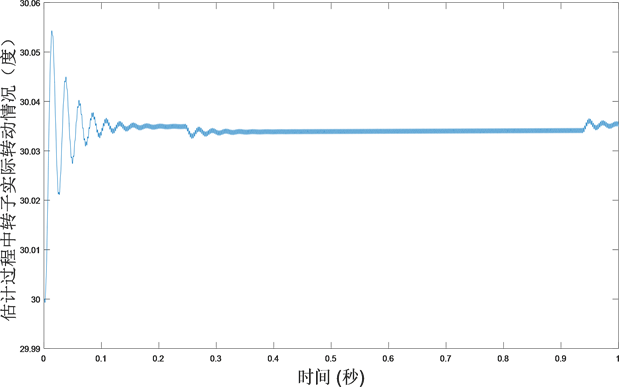Figure 10. PMSM rotor position offset during inspection

5. 结论

 贾洪平, 贺益康. 基于高频注入法的永磁同步电动机转子初始位置检测研究[J]. 中国电机工程学报, 2007, 27(15): 15-20.

 秦峰, 贺益康, 刘毅, 等. 两种高频信号注入法的无传感器运行研究[J]. 中国电机工程学报, 2005, 25(5): 116-121.

 李志强, 夏长亮, 陈炜. 基于线反电动势的无刷直流电机无位置传感器控制[J]. 电工技术学报, 2010, 25(7): 38-44.

 廖勇, 沈朗, 姚骏, 等. 改进的面贴式永磁同步电机转子初始位置检测[J]. 电机与控制学报, 2009, 13(2): 203-207.

 Kim, H., Huh, K.K., Lorenz, R.D., et al. (2004) A Novel Method for Initial Rotor Position Estimation for IPM Synchronous Machine Drives. IEEE Transactions on Industry Applications, 40, 1369-1378.
https://doi.org/10.1109/TIA.2004.834091

 王丽梅, 郭庆鼎. 基于转子凸极跟踪的永磁同步电机转子位置的自检测方法[J]. 电工技术学报, 2001, 16(2): 14-17.

 Jang, G.H., Sul, S.K. and Ha, J.I. (2003) Sensorless Drive of Surface-Mounted Permanent-Magnet Motor by High-Frequency Signal Injection Based on Magnetic Saliency. IEEE Transactions on Industry Applications, 39, 1031-1038.
https://doi.org/10.1109/TIA.2003.813734

 Raca, D., Harke, M.C. and Lorenz, R.D. (2008) Robust Magnet Polarity Estimation for Initialization of PM Synchronous Machines with Near-Zero Saliency. IEEE Transactions on Industry Applications, 44, 1199-1209.
https://doi.org/10.1109/TIA.2008.926195

 王高林, 杨荣峰, 于泳, 等. 内置式永磁同步电机转子初始位置估计方法[J]. 电机与控制学报, 2010, 14(6): 56-60.

 刘颖. 永磁同步电机脉振高频信号注入无位置传感器技术研究[D]: [博士学位论文]. 南京: 南京航空航天大学, 2012.

 王冉珺, 刘恩海. 永磁同步电机转子初始位置的检测方法[J]. 电机与控制学报, 2012, 16(1): 62-66.

 袁雷, 胡冰新, 魏克银, 等. 现代永磁同步电机控制原理及MATLAB仿真[M]. 北京: 北京航空航天大学出版社, 2015: 166-175.

 Jean-Paul Louis, 祝晓辉. 同步电机控制[M]. 北京: 机械工业出版社, 2016: 303-308.

 张海洋, 许海平, 方程, 等. 基于谐振数字滤波器的直驱式永磁同步电机转矩脉动抑制方法[J]. 中国电机工程学报, 2018, 38(4): 1222-1231+1299.

 田士侠. 高频注入法永磁同步电机转子位置检测方法研究[D]: [硕士学位论文]. 济南: 山东大学, 2010.

 金光哲, 徐殿国, 高强, 等. 高频注入电压预估同步电机转子位置检测方法[J]. 中国电机工程学报, 2014, 34(9): 1376-1383.

 贾利娬, 吴帅, 姜忠凯, 等. 永磁同步电机无传感器转子位置观测器研究[J]. 导航与控制, 2015, 14(4): 25-29.

 鲁家栋, 刘景林, 卫丽超. 永磁同步电机转子初始位置检测方法[J]. 电工技术学报, 2015, 30(7): 105-111.

Top